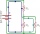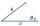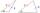# SSA and geometry

The distance between the points P and Q was 356 m measured in the terrain. The PQ line can be seen from the viewer at a viewing angle of 107° 22 '. The observer's distance from P is 271 m. Determine the viewing angle of P and observer.

Result

Q =  46.597 °

#### Solution:Try calculation via our triangle calculator.

Leave us a comment of example and its solution (i.e. if it is still somewhat unclear...):

Showing 0 comments:Be the first to comment!#### To solve this example are needed these knowledge from mathematics:

Need help calculate sum, simplify or multiply fractions? Try our fraction calculator. See also our trigonometric triangle calculator. Try conversion angle units angle degrees, minutes, seconds, radians, grads.

## Next similar examples:

1. Parametric equationFind the parametric equation of a line with y-intercept (0,-4) and a slope of -2.
2. GP membersThe geometric sequence has 10 members. The last two members are 2 and -1. Which member is -1/16?
3. SequenceFind the common ratio of the sequence -3, -1.5, -0.75, -0.375, -0.1875. Ratio write as decimal number rounded to tenth.
4. Effective and mean voltageA voltage divider consisting of resistors R1 = 103000 Ω and R2 = 197000 Ω is connected to the ideal sine wave voltage source, R2 is connected to a voltmeter which measures the mean voltage and has an internal resistance R3 = 200300 Ω, the measured value is
5. A triangleA triangle has an angle that is 63.1 other 2 are in ratio of 2:5 What are the measurements of the two angles?
6. Largest angle of the triangleCalculate the largest angle of the triangle whose sides have the sizes: 2a, 3/2a, 3a
7. MedianThe median of the triangle LMN is away from vertex N 84 cm. Calculate the length of the median, which start at N.
8. Theorem proveWe want to prove the sentense: If the natural number n is divisible by six, then n is divisible by three. From what assumption we started?
9. AnglesIn the triangle ABC, the ratio of angles is: a:b = 4: 5. The angle c is 36°. How big are the angles a, b?
10. Isosceles triangleWhat are the angles of an isosceles triangle ABC if its base is long a=5 m and has an arm b=4 m.
11. Ladder slopeWhat is the slope of a ladder 6.2 m long and 5.12 m in height.
12. Ratio 11Simplify this ratio 10 : 1/4
13. Reference angleFind the reference angle of each angle:
14. Right triangleLadder 16 feet reaches up 14 feet on a house wall. The 90-degree angle at the base of the house and wall. What are the other two angles or the length of the leg of the yard?
15. Angles by cosine lawCalculate the size of the angles of the triangle ABC, if it is given by: a = 3 cm; b = 5 cm; c = 7 cm (use the sine and cosine theorem).
16. RhomboidThe dimensions of the rhomboid sides are a= 5cm, b = 6 cm and the size of the angle at the vertex A is 60°. What is the length of side AC?
17. Two triangles SSATwo triangles can be formed with the given information. Use the Law of Sines to solve the triangles. A = 59°, a = 13, b = 14